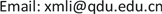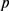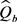﻿ 双参数指数分布的分位数比较 Comparison for Quantiles of Two-Parameter Exponential Distributions

Statistics and Application
Vol. 08  No. 02 ( 2019 ), Article ID: 29560 , 7 pages
10.12677/SA.2019.82027

Comparison for Quantiles of Two-Parameter Exponential Distributions

Shu Liu, Xinmin Li

School of Mathematics, Qingdao University, Qingdao ShandongReceived: Mar. 11th, 2019; accepted: Mar. 26th, 2019; published: Apr. 2nd, 2019ABSTRACT

This paper considers the testing problem for comparing quantiles of the two-parameter exponential distribution. We provide two approaches, the parameters bootstrap and Fiducial method. Simulation studies were conducted to compare two methods. The above methods were also applied in an example.

Keywords:Two-Parameter Exponential Distribution, Quantile, Bootstrap, Fiducial InferenceCopyright © 2019 by authors and Hans Publishers Inc.

This work is licensed under the Creative Commons Attribution International License (CC BY).

http://creativecommons.org/licenses/by/4.0/1. 引言

1989年Tsui和Weerahandi  提出了广义推断的概念。2007年Li，Xu和Li  给出了广义P值的Fiducial推断。1979年Efron  提出的Bootstrap方法应用十分广泛，对于复杂分布的参数和复杂估计可直接导出标准误和区间估计。近年来参数Bootstrap方法也得到了广泛的应用和改进  。本文将分别使用Fiducial推断和参数Bootstrap似然比方法，从假设检验的角度对两个双参数指数分布总体的分位数进行比较。

2. 问题表述

$p\left(x;\mu ,\sigma \right)=\left\{\begin{array}{cc}\begin{array}{l}\frac{1}{\sigma }\mathrm{exp}\left\{-\frac{x-\mu }{\sigma }\right\}\\ 0\end{array}& \begin{array}{l}x>\mu \\ 其他\end{array}\end{array}$

$\left({X}_{i,1},{X}_{i,2},\cdots ,{X}_{i,{n}_{i}}\right)$ 为来自上述分布的容量为 $n$ 的样本，设观测到的数据为定数截断数据为 ${X}_{\left(i1\right)}<{X}_{\left(i2\right)}<\cdots <{X}_{\left(i{r}_{i}\right)}$$i=1,2$。分布 Exp (μI, σi) 的 $p$ 分位数表示为 ${Q}_{{}_{i,p}}={\mu }_{i}-{\sigma }_{i}\mathrm{log}\left(1-p\right)$，从而两个总体分位数的差可写为 ${Q}_{d}={Q}_{2,p}-{Q}_{1,p}$。易知

${W}_{i}={X}_{i,1}$${V}_{i}={\sum }_{j=1}^{{r}_{i}}{X}_{i,j}+\left({n}_{i}-{r}_{i}\right){X}_{i,{r}_{i}}-{n}_{i}{X}_{i,1}$ (1)

${\stackrel{^}{\mu }}_{i}={W}_{i}-\frac{1}{{n}_{i}\left({r}_{i}-1\right)}{V}_{i}$${\stackrel{^}{\sigma }}_{i}=\frac{1}{{r}_{i}-1}{V}_{i}$ (2)

${Q}_{d}$ 的估计为

${\stackrel{^}{Q}}_{d}=\left[{\stackrel{^}{\mu }}_{2}-{\stackrel{^}{\sigma }}_{2}\mathrm{log}\left(1-p\right)\right]-\left[{\stackrel{^}{\mu }}_{1}-{\stackrel{^}{\sigma }}_{1}\mathrm{log}\left(1-p\right)\right]$ (3)

${H}_{0}:{Q}_{d}\le 0\text{ }\text{VS}\text{ }{H}_{1}:{Q}_{d}>0$ (4)

3. 参数Bootstrap方法

Bootstrap方法由Efron  首先提出，其原理是从估计模型中进行重复抽样，常采用的为非参数Bootstrap方法。本文采用参数Bootstrap，用参数的估计取代原参数得到参数模型。

${W}_{i}={E}_{\left(i,1\right)}$${V}_{bi}={\sum }_{j=1}^{{r}_{i}}{E}_{\left(i,j\right)}+\left({n}_{i}-{r}_{i}\right){E}_{\left(i,{r}_{i}\right)}-{n}_{i}{E}_{\left(i,1\right)}$ (5)

${\stackrel{^}{Q}}_{b}=\left[{\stackrel{^}{\mu }}_{b2}-{\stackrel{^}{\sigma }}_{b2}\mathrm{log}\mathrm{log}\left(1-p\right)\right]-\left[{\stackrel{^}{\mu }}_{b1}-{\stackrel{^}{\sigma }}_{b1}\mathrm{log}\left(1-p\right)\right]$ (6)

${\stackrel{^}{\mu }}_{bi}={W}_{bi}-\frac{1}{{n}_{i}\left({r}_{i}-1\right)}{V}_{bi}$${\stackrel{^}{\sigma }}_{bi}=\frac{1}{{r}_{i}-1}{V}_{bi}$$i=1,2$ (7)

(a) 通过(2)式分别计算 ${\stackrel{^}{\mu }}_{i}$${\stackrel{^}{\sigma }}_{i}$

(b) 生成对应的样本容量为 ${r}_{i}$ 的Bootstrap样本并进行排序，

(c) 通过(5) (6) (7)式计算的实现值，

(d) 重复步骤(a)~(c) $N$ 次( $N$ 一般大于1000)，

(e) 给出p值 $p=2\mathrm{min}\left\{\frac{p#\left\{{\stackrel{^}{Q}}_{b}\le 0\right\}}{N},\frac{p#\left\{{\stackrel{^}{Q}}_{b}>0\right\}}{N}\right\}$

4. Fiducial方法

Fiducial推断由Fisher首先提出并研究，这类方法不需要先验分布，而是根据数据给出与参数的后验分布有相同作用的Fiducial分布．其后有不少统计学者对之进行讨论。Hannig等也讨论了利用Fiducial推断方法构造广义区间估计。Li等  给出了利用Fiducial推断进行假设检验的方法。本节利用Fiducial推断研究分位数差的假设检验。

$2{n}_{i}\frac{{W}_{i}-{\mu }_{i}}{{\sigma }_{i}}~{\chi }^{2}\left(2\right)$$\frac{2{V}_{i}}{{\sigma }_{i}}~{\chi }^{2}\left(2{r}_{i}-2\right)$$i=1,2$

${E}_{i,1}~{\chi }^{2}\left(2\right)$${E}_{i,2}~{\chi }^{2}\left(2{r}_{i}-2\right)$ 独立，有

$\left({W}_{i},{V}_{i}\right)=\left(\frac{{\sigma }_{i}{E}_{i,1}}{2{n}_{i}}+{\mu }_{i},\frac{{\sigma }_{i}{E}_{i,2}}{2}\right)$

$\left({\mu }_{i},{\sigma }_{i}\right)=\left({w}_{i}-\frac{{v}_{i}{e}_{1}}{{n}_{i}{e}_{2}},\frac{2{v}_{i}}{{e}_{2}}\right)$

${T}_{{\mu }_{i}}={w}_{i}-\frac{{v}_{i}{E}_{1}}{{n}_{i}{E}_{2}},{T}_{{\sigma }_{i}}=\frac{2{v}_{i}}{{E}_{2}}$

${T}_{{Q}_{d}}=\left[{T}_{{\mu }_{2}}-{T}_{{\sigma }_{2}}\mathrm{log}\left(1-p\right)\right]-\left[{T}_{{\mu }_{1}}-{T}_{{\sigma }_{1}}\mathrm{log}\left(1-p\right)\right]$

$p=2\mathrm{min}\left\{p\left({T}_{{Q}_{d}}<0\right),p\left({T}_{{Q}_{d}}>0\right)\right\}$

1) 对给定数据，选取一个较大的模拟样本数N，

2) 对于 $j=1,\cdots ,N$，通过 ${E}_{i}$ 的分布 $Exp\left({\mu }_{i},{\sigma }_{i}\right)$ 随机生成 ${e}_{j}$

3) 求方程 $\left({w}_{i},{v}_{i}\right)=\left(\frac{{\sigma }_{i}{e}_{i,1}}{2{n}_{i}}+{\mu }_{i},\frac{{\sigma }_{i}{e}_{i,2}}{2}\right)$ 的解并计算 ${T}_{{Q}_{d}}$ 的实现值 ${T}_{i}$${T}_{{Q}_{d}}=\left[{T}_{{\mu }_{2}}-{T}_{{\sigma }_{2}}\mathrm{log}\left(1-p\right)\right]-\left[{T}_{{\mu }_{1}}-{T}_{{\sigma }_{1}}\mathrm{log}\left(1-p\right)\right]$

4) 计算p值 $p=2\mathrm{min}\left\{\frac{p#\left\{{T}_{i}<0\right\}}{N},\frac{p#\left\{{T}_{i}>0\right\}}{N}\right\}$

5. 数据仿真模拟

Table 1. Power of two methods (n = 10, r = 8)

Table 2. Power of two methods (n = 50, r = 40)

6. 实例分析

7. 结论

Comparison for Quantiles of Two-Parameter Exponential Distributions[J]. 统计学与应用, 2019, 08(02): 244-250. https://doi.org/10.12677/SA.2019.82027

1. 1. Lawless, J.F. (1983) Statistical Methods in Reliability. Journal of the American Statistical Association, 25, 305-316.

2. 2. Roy, A. and Mathew, T. (2005) A Generalized Confidence Limit for the Reliability Function of a Two-Parameter Exponential Distribution. Journal of Statistical Planning and Inference, 128, 509-517.

3. 3. Wright, F.T., Engelhard, T. and Bain, M.L.J. (1978) Inferences for the Two-Parameter Exponential Distribution under Type I Censored Sampling. Journal of the American Statistical Association, 73, 650-655. https://doi.org/10.1080/01621459.1978.10480072

4. 4. Fernández, A.J. (2007) On Calculating Generalized Con-fidence Intervals for the Two-Parameter Exponential Reliability Function. Annals of Statistics, 41, 129-135. https://doi.org/10.1080/10485250601033172

5. 5. Tsui, K.W. and Weerahandi, S. (1989) Generalized P-Values in Significance Testing of Hypotheses in the Presence of Nuisance Parameters. Journal of the American Statistical Asso-ciation, 84, 602-607.

6. 6. Li, X., Xu, X. and Li, G. (2007) A Fiducial Argument for Generalized P-Value. Science in China Series A: Mathematics, 50, 957-966. https://doi.org/10.1007/s11425-007-0067-7

7. 7. Efron, B. (1979) Bootstrap Methods: Another Look at the Jackknife. Annals of Statistics, 7, 1-26. https://doi.org/10.1214/aos/1176344552

8. 8. Tian, L.L., Ma, C.X. and Vexler, A. (2009) A Parametric Bootstrap Test for Comparing Heteroscedastic Regression Models. Communications in Statistics-Simulation and Computation, 38, 1026-1036. https://doi.org/10.1080/03610910902737077

9. 9. Bain, L.J. (1978) Statistical Analysis of Reliability and Life-Testing Models. Marcel Dekker, New York, NY.